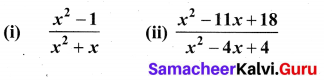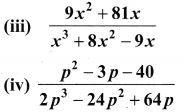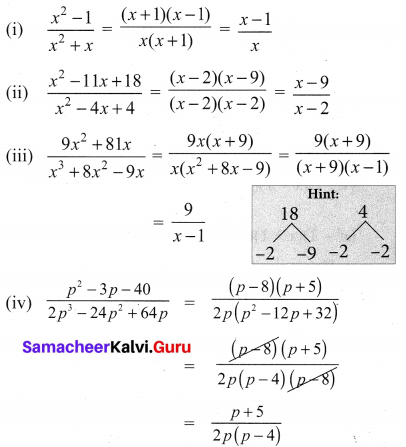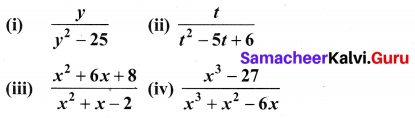## Tamilnadu Samacheer Kalvi 10th Maths Solutions Chapter 3 Algebra Ex 3.4

10th Maths Exercise 3.4 Samacheer Kalvi Question 1.
Reduce each of the following rational expressions to its lowest form.Solution:Ex 3.4 Class 10 Samacheer Question 2.
Find the excluded values, if any of the following expressions.Solution:
(i) $$\frac{y}{y^{2}-25}=\frac{y}{(y+5)(y-5)}$$ is undefined when (y + 5) (y – 5) = 0 that is y = -5, 5
∴ The excluded values are -5, 5

(ii) $$\frac{t}{t^{2}-5 t+6}$$ is undefined when t2 – 5t + 6 = 0 i.e.
(t – 3) (t – 2) = 0 ⇒ t = 3, 2
∴ The excluded values are 3, 2

(iii) $$\frac{x^{2}+6 x+8}{x^{2}+x-2}$$ is undefined when x2 + x – 2 = 0 i.e.
(x + 2) (x + 1) = 0
∴ The excluded values are 2, 1

(iv) $$\frac{x^{3}-27}{x^{3}+x^{2}-6 x}$$ is undefined when x3 + x2 – 6x = 0, i.e
x(x2 + x – 6) = 0
x(x + 3) (x – 2) = 0
∴ The excluded values are -3, 2